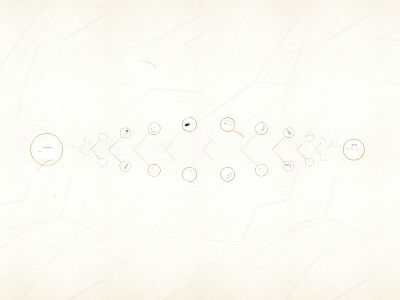Publications: 51 | Followers: 2

## Cryptography - cs.umd.edu

Publish on Category: Birds 0

Cryptography
Lecture 23
Cyclic groups
Let G be a finite group of orderq(written multiplicatively)Let g be some element of GConsider the set <g> = {g0, g1, …}We knowgq= 1 = g0, so the set has ≤qelementsIf the set hasqelements, then it is all of G !In this case, we say g is ageneratorof GIf G has a generator, we say G iscyclic
Examples
ℤNCyclic (for any N); 1 is always a generator:{0, 1, 2, …, N-1}ℤ8Is 3 a generator?{0, 3, 6, 1, 4, 7, 2, 5} – yes!Is 2 a generator?{0, 2, 4, 6} – no!
Example
ℤ*11Is 3 a generator?{1, 3, 9, 5, 4} – no!Is 2 a generator?{1, 2, 4, 8, 5, 10, 9, 7, 3, 6} – yes!Is 8 a generator?{1, 8, 9, 6, 4, 10, 3, 2, 5, 7} – yes!Note that elements appear in a different order from above…
Example
ℤ*13<2> = {1, 2, 4, 8, 3, 6, 12, 11, 9, 5, 10, 7},so 2 is a generator<8> = {1, 8, 12, 5},so 8 is not a generator
Important examples
Theorem:Any group of prime order is cyclic, and every non-identity element is a generatorTheorem:If p is prime, thenℤ*pis cyclicNote: the order is p-1, which is not prime for p > 3
Uniform sampling
Given cyclic group G of order q along with generator g, easy to sample a uniformhG:Choose uniform x{0, …, q-1}; set h :=gx
Discrete-logarithm problem
Fix cyclic group G of order q, and generator gWe know that {g0, g1, …, gq-1} = GFor everyhG, there is a uniquexℤqs.t.gx= hDefinelogghto be this x –the discrete logarithmof h with respect to g(in the group G)
Examples
Inℤ*11What is log29?<2> ={1, 2, 4, 8, 5, 10, 9, 7, 3,6},so log29 = 6What is log89?<8> ={1, 8, 9, 6, 4, 10, 3, 2, 5,7}, so log89 = 2Inℤ*13What is log29?<2> ={1, 2, 4, 8, 3, 6, 12, 11, 9, 5, 10,7}, so log29 = 8
Discrete-logarithm problem (informal)
Dlogproblem in G:Givengenerator g and element h, computelogghDlogassumption in G:Solving the discrete log problem in G ishard
Example
Inℤ*3092091139What is log21656755742 ?
Discrete-logarithm problem
LetGbe a group-generation algorithmOn input 1n, outputs a(description of a) cyclicgroup G, its order q (withǁqǁ=n), and a generator gFor algorithm A, defineexp’tDlogA,G(n):Compute (G, q, g)G(1n)Choose uniformhGRun A(G, q, g, h) to get xExperiment evaluates to 1 ifgx= h
Discrete-logarithm problem
Thediscrete-logarithm problem is hard relative toGif for all PPT algorithms A,Pr[DlogA,G(n) = 1]≤negl(n)
Diffie-Hellman problems
Fix cyclic group G and generator gDefineDHg(h1, h2) =DHg(gx,gy) =gxy
Example
Inℤ*11<2> ={1, 2, 4, 8, 5, 10, 9, 7, 3, 6}So DH2(7, 5) = ?Inℤ*3092091139What is DH2(1656755742,938640663)?Is 1994993011 the answer, or is it just a random element ofℤ*3092091139?
Diffie-Hellman assumptions
ComputationalDiffie-Hellman (CDH) problem:Given g, h1, h2, computeDHg(h1, h2)DecisionalDiffie-Hellman (DDH) problem:Given g, h1, h2, distinguishDHg(h1, h2) from a uniform element of G
DDH problem
LetGbe a group-generation algorithmOn input 1n, outputs a cyclic group G, its order q (withǁqǁ=n), and a generator gThe DDH problem is hard relative toGif for all PPT algorithms A:|Pr[A(G, q, g,gx,gy,gz)=1] –Pr[A(G, q, g,gx,gy,gxy)=1] | ≤(n)
Relating theDiffie-Hellman problems
Relative toG:If the discrete-logarithm problem is easy, so is the CDH problemIf the CDH problem is easy, so is the DDH problemI.e., the DDH assumption isstrongerthan the CDH assumptionI.e., the CDHassumption isstrongerthan thedlogassumption
Group selection
The discrete logarithm is not hard in all groups!For example, it is easy inℤN(for any N, and for any generator)Nevertheless, there are certain groups where the problem is believed to be hardNote: since all cyclic groups of the same order are isomorphic, the group representation matters!
Group selection
For cryptographic applications, best to useprime-ordergroupsThedlogproblem becomes easier if the order of the group has small prime factorsPrime-order groups have several nice featuresE.g., every element except identity is a generatorTwo common choices of groups…
Group selection: choice 1
Prime-order subgroup ofℤ*p, p primeE.g., p =tq+ 1 for q primeTake the subgroup oftthpowers, i.e.,G = { [xtmod p]| xℤ*p}This is a groupIt has order (p-1)/t = qSince q is prime, the group must be cyclicGeneralizations based on finite fields also used
Group selection: choice 2
Prime-order subgroup of anelliptic curvegroupSee book for details…
Group selection
We will describealgorithms in “abstract” groupsCan ignore details of the underlying group in the analysisCan instantiate with any (appropriate) group for animplementation

0

Embed

Share

Upload

Make amazing presentation for free
Cryptography - cs.umd.edu